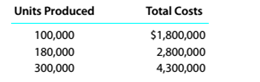Chapter 11, Problem 11.7E

Chapter
Section
Textbook Problem

High-low method Liberty Inc. has decided to use the high-low method to estimate costs. The data for various levels of production are as follows:a. Determine the variable cost per unit and the fixed cost. b.Based on part (a), estimate the total cost for 260.000 units of production.

To determine

Concept Introduction:

Mixed Costs:

There are three types of costs according to the unit of production; Variable, Fixed and Mixed. Variable costs change proportionally with the number of units produced and variable cost per unit remains constant. Fixed Cost remains same in totality irrespective of the number of units produced.

The mixed cost is the mix of variable and fixed cost, some of its part is fixed and some variable.

High-Low Method:

This method is used to determine the fixed as well as variable part of the mixed cost. The formula for calculation of variable cost per unit is as follows:

Variable cost per unit =  ( High Cost - Low Cost ) ( High units - low units )

The formula to calculate the Fixed Cost is as follows:

Fixed Cost = Total cost - (Units×Variable cost per unit)

Requirement-1:

To Calculate:

The variable cost per unit and fixed cost per unit

Explanation

The variable cost per unit and fixed cost per unit are as follows:

 High Low Method High Low Difference A B A-B Number of units 300000
To determine

Concept Introduction:

Mixed Costs:

There are three types of costs according to the unit of production; Variable, Fixed and Mixed. Variable costs change proportionally with the number of units produced and variable cost per unit remains constant. Fixed Cost remains same in totality irrespective of the number of units produced.

The mixed cost is the mix of variable and fixed cost, some of its part is fixed and some variable.

High-Low Method:

This method is used to determine the fixed as well as variable part of the mixed cost. The formula for calculation of variable cost per unit is as follows:

Variable cost per unit =  ( High Cost - Low Cost ) ( High units - low units )

The formula to calculate the Fixed Cost is as follows:

Fixed Cost = Total cost - (Units×Variable cost per unit)

Requirement-2:

To Calculate:

The Cost of 260,000 units

Still sussing out bartleby?

Check out a sample textbook solution.

See a sample solution

The Solution to Your Study Problems

Bartleby provides explanations to thousands of textbook problems written by our experts, many with advanced degrees!

Get Started

Select the independent and dependent variables.

Managerial Accounting: The Cornerstone of Business Decision-Making

Would it ever be rational for a firm to borrow money in order to pay cash dividends? Explain.

Fundamentals of Financial Management, Concise Edition (with Thomson ONE - Business School Edition, 1 term (6 months) Printed Access Card) (MindTap Course List)

(Oligopoly Power) What are three sources of oligopolies?

ECON: MICRO4 (New, Engaging Titles from 4LTR Press)